Topics: AI | Data Science | Data Visualization | Deep Learning | Machine Learning | NLP | Python | R | Statistics

KDnuggets Home » News » 2017 » Aug » Tutorials, Overviews » Support Vector Machine (SVM) Tutorial: Learning SVMs From Examples

# Support Vector Machine (SVM) Tutorial: Learning SVMs From Examples

http likes 143

In this post, we will try to gain a high-level understanding of how SVMs work. I’ll focus on developing intuition rather than rigor. What that essentially means is we will skip as much of the math as possible and develop a strong intuition of the working principle.

### Kernels

Finally, the secret sauce that makes SVMs tick. This is where we need to look at a bit of math.

Let’s take stock of what we have seen so far:

1. For linearly separable data SVMs work amazingly well.
2. For data that’s almost linearly separable, SVMs can still be made to work pretty well by using the right value of C.
3. For data that’s not linearly separable, we can project data to a space where it is perfectly/almost linearly separable, which reduces the problem to 1 or 2 and we are back in business.

It looks like a big part of what makes SVMs universally applicable is projecting it to higher dimensions. And this is where kernels come in.

First, a slight digression.

A very surprising aspect of SVMs is that in all of the mathematical machinery it uses, the exact projection, or even the number of dimensions, doesn’t show up. You could write all of it in terms of the dot productsbetween various data points (represented as vectors). For p-dimensional vectors i and j where the first subscript on a dimension identifies the point and the second indicates the dimension number: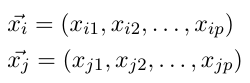The dot product is defined as: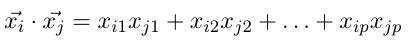If we have n points in our dataset, the SVM needs only the dot product of each pair of points to find a classifier. Just that. This is also true when we want to project data to higher dimensions. We don’t need to provide the SVM with exact projections; we need to give it the dot product between all pairs of points in the projected space.

This is relevant because this is exactly what kernels do. A kernel, short for kernel function, takes as input two points in the original space, and directly gives us the dot product in the projected space.
Let’s revisit the projection we did before, and see if we can come up with a corresponding kernel. We will also track the number of computations we need to perform for the projection and then finding the dot products — to see how using a kernel compares.

For a point i: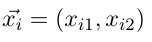Our corresponding projected point was: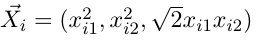To compute this projection we need to perform the following operations:

• To get the new first dimension: 1 multiplication
• Second dimension: 1 multiplication
• Third dimension: 2 multiplications

In all, 1+1+2 = 4 multiplications.

The dot product in the new dimension is: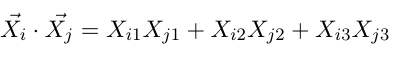To compute this dot product for two points and j, we need to compute their projections first. So that’s 4+4 = 8 multiplications, and then the dot product itself requires 3 multiplications and 2 additions.

In all, that’s:

• Multiplications: 8 (for the projections) + 3 (in the dot product) = 11 multiplications
• Additions: 2 (in the dot product)

Which is total of 11 + 2 = 13 operations.

I claim this kernel function gives me the same result: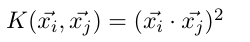We take the dot product of the vectors in the original space first, and then square the result.

Let expand it out and check whether my claim is indeed true: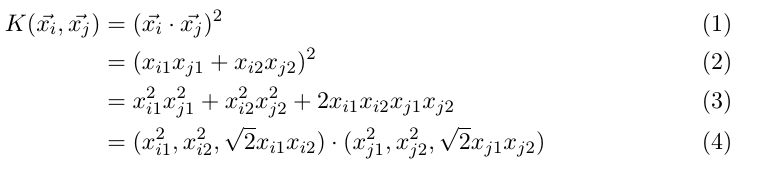It is. How many operations does this need? Look at step (2) above. To compute the dot product in two dimensions I need 2 multiplications and 1 addition. Squaring it is another multiplication.

So, in all:

• Multiplications: 2 (for the dot product in the original space) + 1 (for squaring the result) = 3 multiplications
• Additions: 1 (for the dot product in the original space)

A total of 3 + 1 = 4 operations. This is only 31% of the operations we needed before.

It looks like it is faster to use a kernel function to compute the dot products we need. It might not seem like a big deal here: we’re looking at 4 vs 13 operations, but with input points with a lot more dimensions, and with the projected space having an even higher number of dimensions, the computational savings for a large dataset add up incredibly fast. So that’s one huge advantage of using kernels.

Most SVM libraries already come pre-packaged with some popular kernels like Polynomial, Radial Basis Function (RBF), and Sigmoid. When we don’t use a projection (as in our first example in this article), we compute the dot products in the original space — this we refer to as using the linear kernel.

Many of these kernels give you additional levers to further tune it for your data. For example, the polynomial kernel: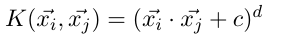allows you to pick the value of and d (the degree of the polynomial). For the 3D projection above, I had used a polynomial kernel with c=0 and d=2.

But we are not done with the awesomeness of kernels yet!

Remember I mentioned projecting to infinite dimensions a while back? If you haven’t already guessed, the way to make it work is to have the right kernel function. That way, we really don’t have to project the input data, or worry about storing infinite dimensions.

A kernel function computes what the dot product would be if you had actually projected the data.

The RBF kernel is commonly used for a specific infinite-dimensional projection. We won’t go into the math of it here, but look at the references at the end of this article.

How can we have infinite dimensions, but can still compute the dot product? If you find this question confusing, think about how we compute sums of infinite series. This is similar. There are infinite terms in the dot product, but there happens to exist a formula to calculate their sum.

1. We typically don’t define a specific projection for our data. Instead, we pick from available kernels, tweaking them in some cases, to find one best suited to the data.
2. Of course, nothing stops us from defining our own kernels, or performing the projection ourselves, but in many cases we don’t need to. Or we at least start by trying out what’s already available.
3. If there is a kernel available for the projection we want, we prefer to use the kernel, because that’s often faster.
4. RBF kernels can project points to infinite dimensions.

### SVM libraries to get started

There are quite a few SVM libraries you could start practicing with:

Many general ML libraries like scikit-learn also offer SVM modules, which are often wrappers around dedicated SVM libraries. My recommendation is to start out with the tried and tested libSVM.

libSVM is available as a commandline tool, but the download also bundles Python, Java, and Matlab wrappers. As long as you have a file with your data in a format libSVM understands (the README that’s part of the download explains this, along with other available options) you are good to go.

In fact, if you need a really quick feel of how different kernels, the value of C, etc., influence finding the separating boundary, try out the “Graphical Interface” on their home page. Mark your points for different classes, pick the SVM parameters, and hit Run!

I couldn’t resist and quickly marked a few points: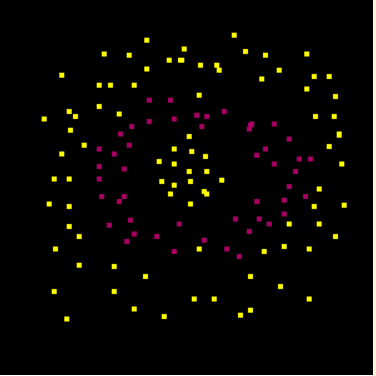Yep, I’m not making it easy for the SVM.

Then I tried out a couple of kernels: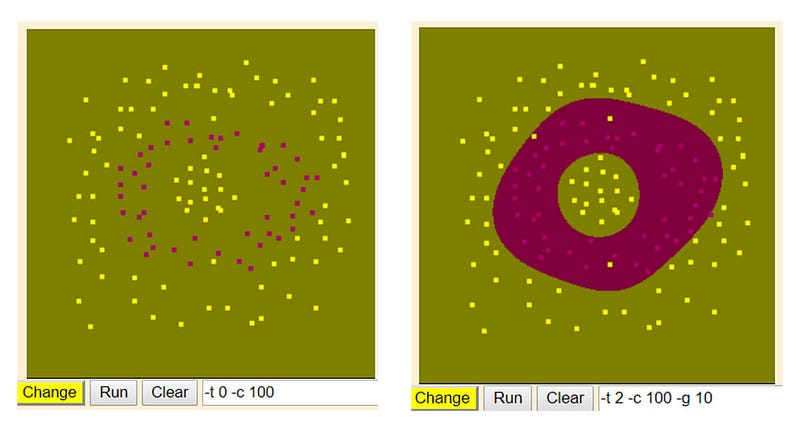The interface doesn’t show you the separating boundary, but shows you the regions that the SVM learns as belonging to a specific label. As you can see, the linear kernel completely ignores the red points. It thinks of the whole space as yellow (-ish green). But the RBF kernel neatly carves out a ring for the red label!

We have been primarily relying on visual intuitions here. While that’s a great way to gain an initial understanding, I’d strongly encourage you to dig deeper. An example of where visual intuition might prove to be insufficient is in understanding margin width and support vectors for non-linearly separable cases.

Remember that these quantities are decided by optimizing a trade-off. Unless you look at the math, some of the results may seem counter-intuitive.

Another area where getting to know the math helps is in understanding kernel functions. Consider the RBF kernel, which I’ve barely introduced in this short article. I hope the “mystique” surrounding it — its relation to an infinite-dimensional projection coupled with the fantastic results on the last dataset (the “ring”) — has convinced you to take a closer look at it.

Resources I would recommend:

1. Video Lectures: Learning from Data by Yaser Abu-Mostafa. Lectures from 14 to 16 talk about SVMs and kernels. I’d also highly recommend the whole series if you’re looking for an introduction to ML, it maintains an excellent balance between math and intuition.
2. Book: The Elements of Statistical Learning — Trevor Hastie, Robert Tibshirani, Jerome Friedman.Chapter 4 introduces the basic idea behind SVMs, while Chapter 12 deals with it comprehensively.

Happy (Machine) Learning!

Bio: Abhishek Ghose is a Senior Data Scientist at 24/7, Inc.

Original. Reposted with permission.

Related:Get KDnuggets, a leading newsletter on AI, Data Science, and Machine Learning